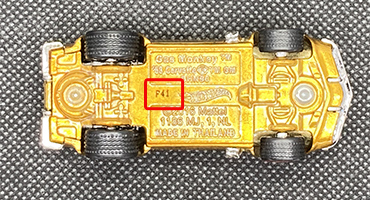• Osseo, Minnesota

## Mattel Hot Wheels Date (base) Codes

What is Hot Wheels date code?

Starting in 2008, Mattel introduced a manufacture date code on the bottom of Hot Wheels and Matchbox - refer to as the Base Code. The first character is a letter that represents the year and the numbers which represent which week of the year the car was manufactured.

For example, if you have a base code that reads "B30", that is a casting from the 30th week of 2009. A base code reading "P41" is a casting from the 41th week of 2021 (the week of October 10, 2021). Letter "I", "O", & "Q" are not used.

Base Codes Letters• A = 2008
• B = 2009
• C = 2010
• D = 2011
• E = 2012
• F = 2013
• G = 2014
• H = 2015
• J = 2016
• K = 2017
• L = 2018
• M = 2019
• N = 2020
• P = 2021
• R = 2022
• S = 2023
• T = 2024
• U = 2025
• V = 2026
• W = 2027
• X = 2028
• Y = 2029
• Z = 2030

### Hot Wheels Check List